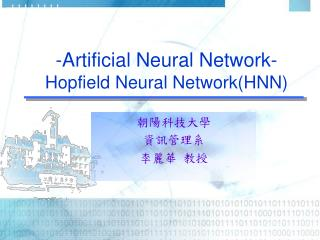DownloadDownload Presentation-Artificial Neural Network- Hopfield Neural Network(HNN)

# -Artificial Neural Network- Hopfield Neural Network(HNN)

Download Presentation## -Artificial Neural Network- Hopfield Neural Network(HNN)

- - - - - - - - - - - - - - - - - - - - - - - - - - - E N D - - - - - - - - - - - - - - - - - - - - - - - - - - -
##### Presentation Transcript

1. -Artificial Neural Network- Hopfield Neural Network(HNN) 朝陽科技大學 資訊管理系 李麗華 教授

2. Assoicative Memory (AM) -1 • Def:Associative memory (AM) is any device that associates a set of predefined output patterns with specific input patterns. • Two types of AM: • Auto-associative Memory: Converts a corrupted input pattern into the most resembled input. • Hetro-associative Memory: Produces an output pattern that was stored corresponding to the most similar input pattern.

3. v1 v2 v3 : vm Assoicative Memory X1 X2 X3 : Xn Assoicative Memory (AM) - 2 Models: It is the associative mapping of an input vector X into the output vector V. EX: Hopfield Neural Network (HNN) EX: Bidirectional Associative Memory (BAM)

4. … X2 X1 Xn Introduction • Hopfield Neural Network(HNN) was proposed by Hopfield in 1982. • HNN is an auto-associative memory network. • It is a one layer, fully connected network.

5. … +1 net j > 0 Xi if netj = 0 -1 net j < 0 X1 X2 Xn HNN Architecture • Input： Xi ﹛-1, +1﹜ • Output：same as input(∵single layer network) • Transfer function：Xi new= • Weights： • Connections： (Xi是指前一個X值)

6. HNN Learning Process • Learning Process： a. Setup the network, i.e., design the input nodes & connections. b. Calculate and derived the weight matrix C. Store the weight matrix. The learning process is done when the weight matrix is derived. We shall obtain a nxn weight matrix, Wnxn.

7. （or net = W‧X i） HNN Recall Process • Recall a. Read the nxn weight matrix, Wnxn. b. Input the test pattern X for recalling. c. Compute new input （i.e. output） d. Repeat process c. until the network converge （i.e. the net value is not changed or the error is very small） +1 net j > 0 Xj old if net j = 0 +1 net j < 0 X j： X new

8. Example: Use HNN to memorize patterns (1) • Use HNN to memorize the following patterns. Let the Green color is represented by “1” and white color is represented by “-1”. The input data is as shown in the table X3 X4 X2 X1

9. Example: Use HNN to memorize patterns (3) Recall The pattern is recalled as:

10. -Artificial Neural Network-Bidirectional Associative Memory (BAM) 朝陽科技大學 資訊管理系 李麗華 教授

11. Ym Y2 ‧‧‧‧‧‧ Y1 ‧‧‧‧‧‧‧ Introduction • Bidirectional Associative Memory (BAM) was proposed by Bart Kosko in 1985. • It is a hetro-associative memory network. • It allows the network to memorize from a set of pattern Xp to recall another set of pattern Yp

12. Assoicative Memory (AM) 1 • Def:Associative memory (AM) is any device that associates a set of predefined output patterns with specific input patterns. • Two types of AM: • Auto-associative Memory: Converts a corrupted input pattern into the most resembled input. • Hetro-associative Memory: Produces an output pattern that was stored corresponding to the most similar input pattern.

13. v1 v2 v3 : vm Assoicative Memory X1 X2 X3 : Xn Assoicative Memory (AM) 2 Models: It is the associative mapping of an input vector X into the output vector V. EX: Hopfield Neural Network (HNN) EX: Bidirectional Associative Memory (BAM)

14. Ym Y2 ‧‧‧‧‧‧ Y1 ‧‧‧‧‧‧‧ BAM Architecture • Input layer： • Output layer： • Weights： • Connection： It’s a 2-layer, fully connected, feed forward & feed back network.

15. BAM Architecture (cont.) • Transfer function：

16. BAM Example(1/4) ● ○● ○● ○ ○●○●○● ● ●● ● ●● ○ ○○ ○ ○○ ●●● ○●○ Test pattern

17. BAM Example(2/4) 1. Learning • Set up network • Setup weights

18. BAM Example(3/4) 2. Recall • Read network weights • Read test pattern • Compute Y • Compute X • Repeat (3) & (4) until converge

19. BAM Example(4/4) • 聚類之Application test pattern (1 1 1 -1 1 -1)1*6 (1) (2) 二次都相同 ●●● ○●○ 